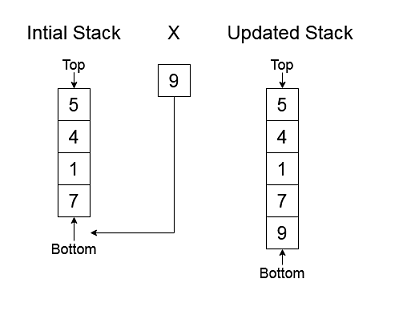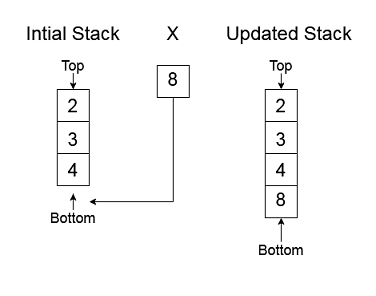Problem title
Difficulty
Avg time to solve

Shortest Substring with all characters
Easy
--
Left View Of a Binary Tree
Easy
10 mins
Roman Numeral To Integer
Easy
15 mins
Maximum length pair chain
Moderate
15 mins
Optimal BST
Hard
55 mins
Regular Expression Matching
Hard
25 mins
Rabin Carp
Moderate
10 mins
Insert An Element At Its Bottom In A Given Stack
Easy
15 mins
Preorder Traversal
Easy
15 mins
Print All Subsets
Easy
20 mins16

# Insert An Element At Its Bottom In A Given Stack

Difficulty: EASY
Avg. time to solve
15 min
Success Rate
85%

Problem Statement

#### You are given a stack/deque of integers 'MY-STACK' and an integer ‘X’. Your task is to insert ‘X’ to the bottom of ‘MY-STACK’ and return the updated stack/deque.

##### Note :
``````If ‘MY_STACK’ = [7, 1, 4, 5], then the first element represents the element at the bottom of the stack and the last element represents the element at the top of the stack.
``````
##### For Example :
``````Let ‘MY_STACK’ = [7, 1, 4, 5] and ‘X’ = 9. So, ‘MY_STACK’ after insertion becomes [9, 7, 1, 4, 5].
````````````Try to do this without using any other data structure.
``````
##### Input Format :
``````The first line of input contains a single integer T’, representing the number of test cases.

The first line of each test case contains two single space-separated numbers, ‘N’ and ‘X’, denoting the size of ‘MY_STACK’ and the integer to be inserted at the bottom, respectively.

The second line contains ‘N’ space-separated distinct integers denoting the stack/deque elements.
``````
##### Output Format :
``````For each test case, print the elements of the updated ‘MY_STACK’ separated by a single space.

The output of every test case will be printed in a separate line.
``````
##### Note :
``````You don’t have to print anything, it has already been taken care of. Just implement the given function.
``````
##### Constraints :
``````1 <= T <=100
1 <= N <= 10^4
0 <= 'X’ and 'MY_STACK[i]’ <= 10^5

Time limit: 1 second
``````
##### Sample input 1 :
``````2
3 8
4 3 2
4 5
6 2 4 9
``````
##### Sample Output 1 :
``````8 4 3 2
5 6 2 4 9
``````
##### Explanation For Sample Input 1 :
``````Test Case 1:
````````````We are  given 'MY_STACK = [4,3,2] and 'X’ = 8.
We insert ‘X’ at the bottom of ‘MY_STACK’.
So finally, we have 'MY_STACK' = [8, 4, 3, 2].

Test Case 2:
After inserting an element at its bottom, the stack will be 5 6 2 4 9
``````
##### Sample Input 2 :
``````2
1 0
4
2 5
1 9
``````
##### Sample Output 2 :
``````0 4
5 1 9
``````
##### Explanation For Sample Input 2 :
``````Test Case 1:
We are given 'MY_STACK = ’ and 'X’ = 0’.
We insert ‘X’ at the bottom of ‘MY_STACK’.
So finally, 'MY_STACK' will become [0, 4].

Test Case 2:
We are given 'MY_STACK = [1,9]’ and 'X’ = 5.
So finally, 'MY_STACK' will become [5, 1, 9].
``````Console